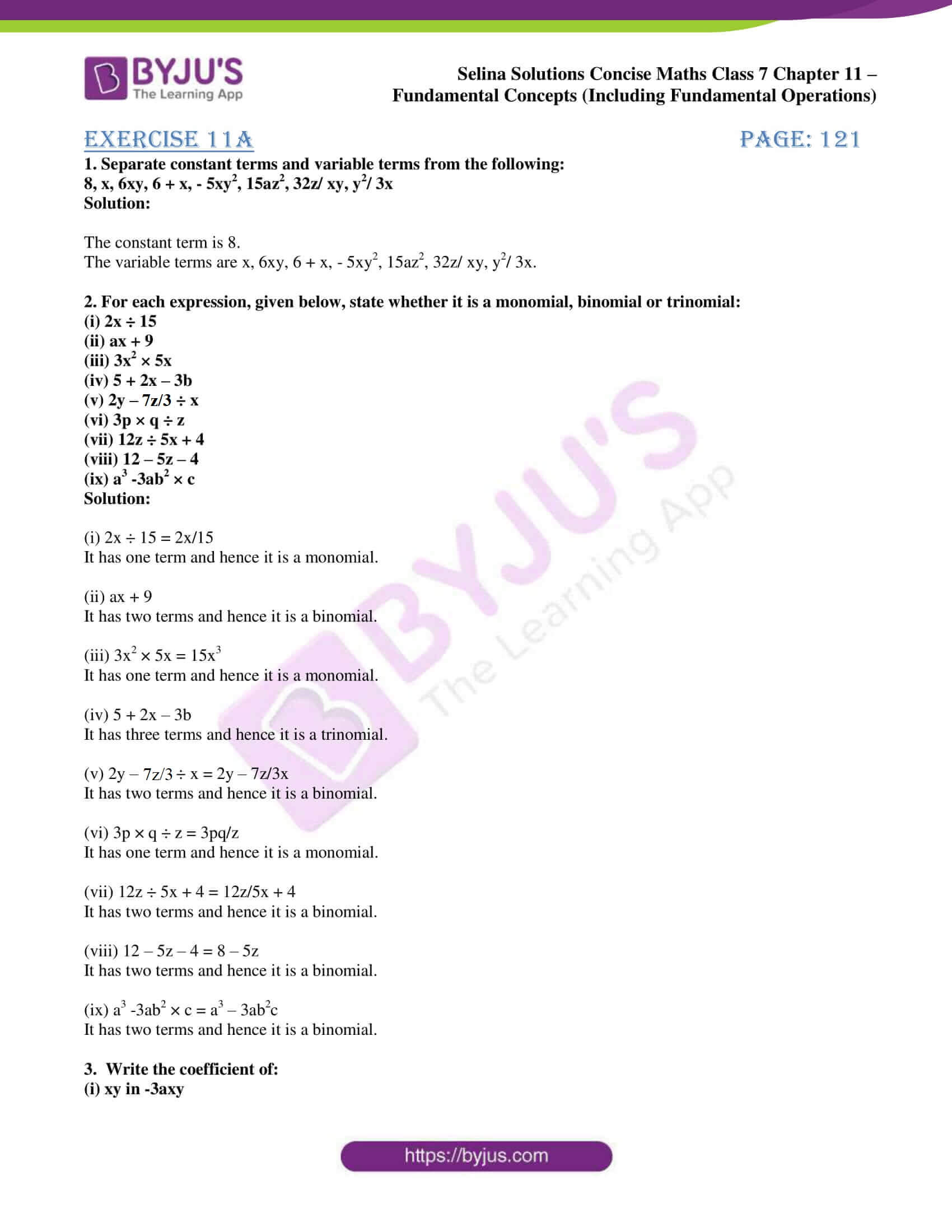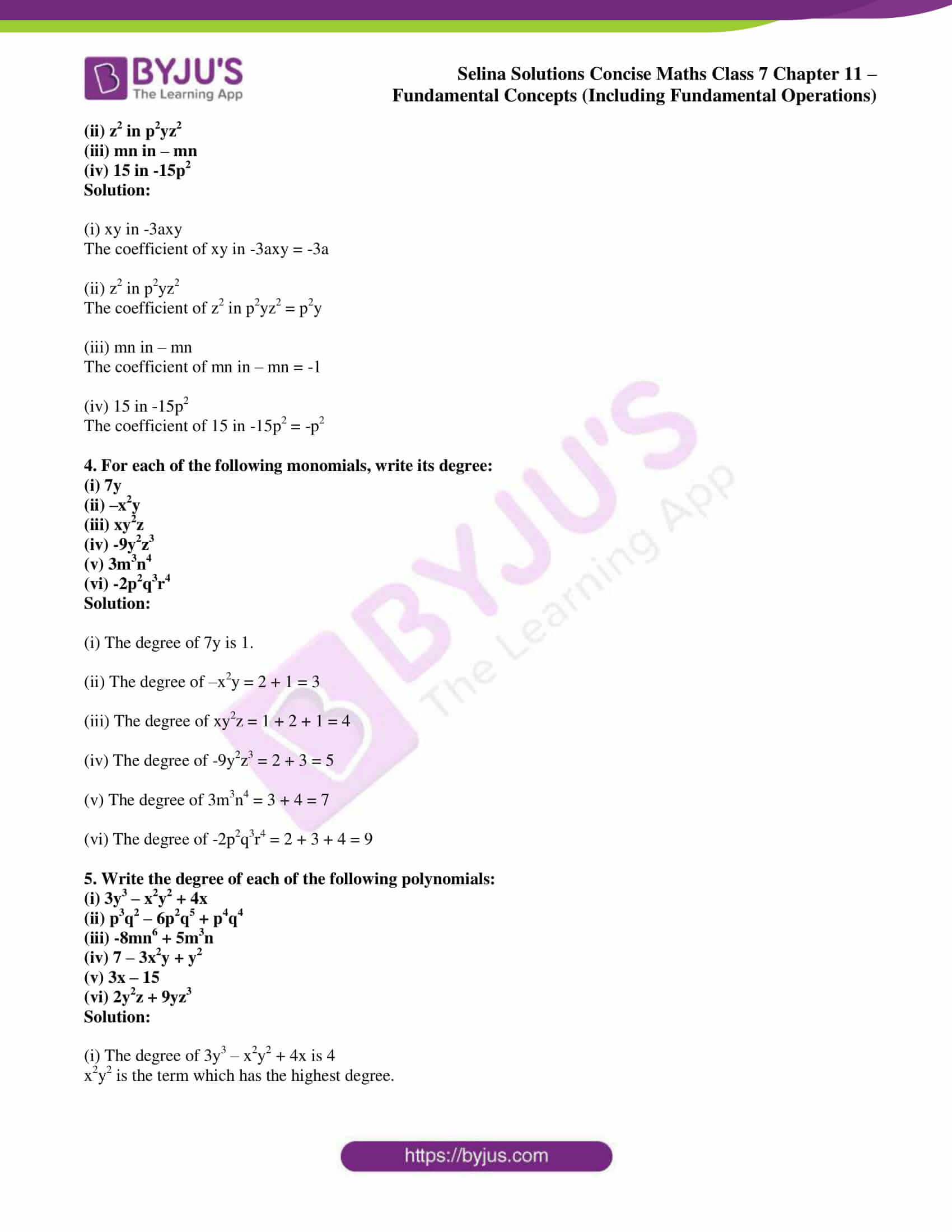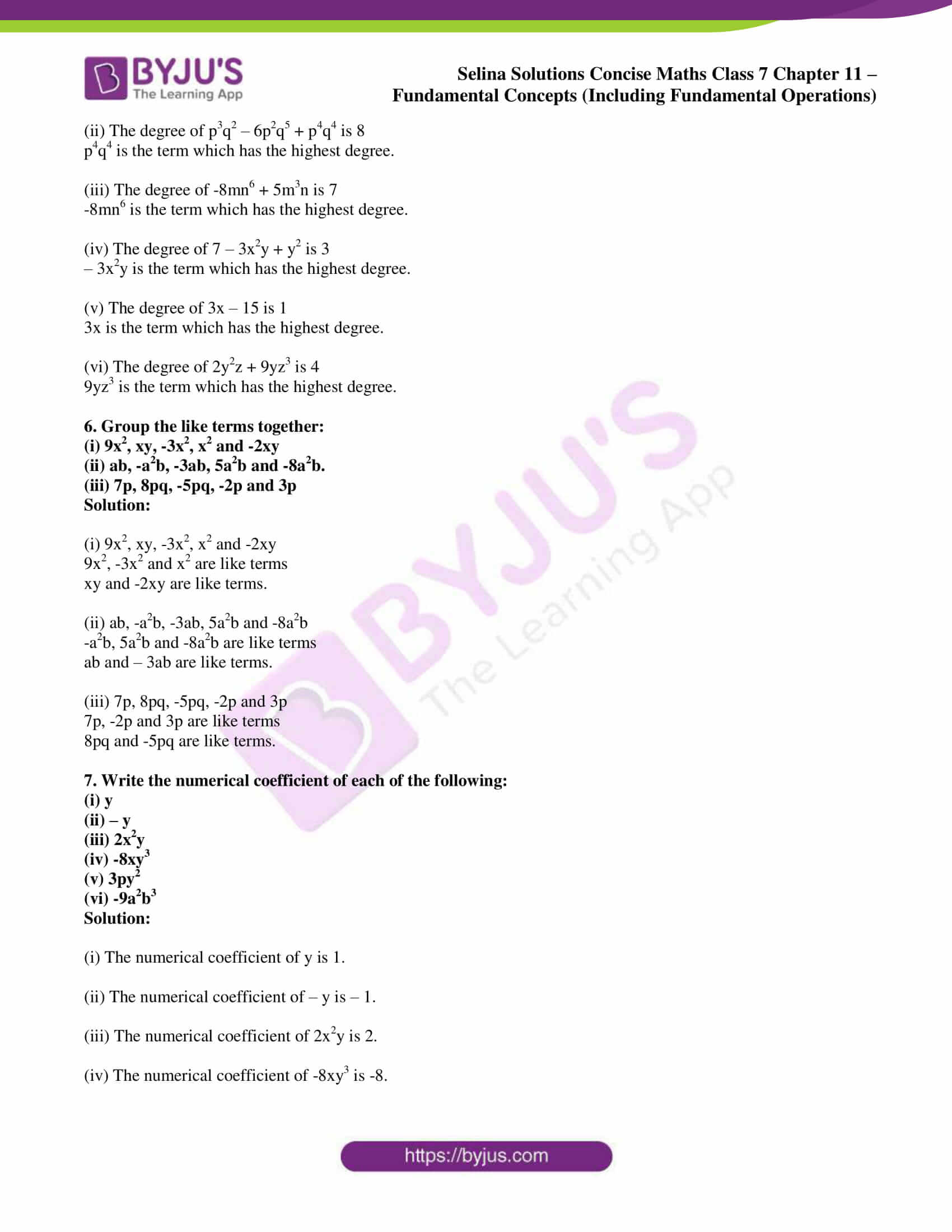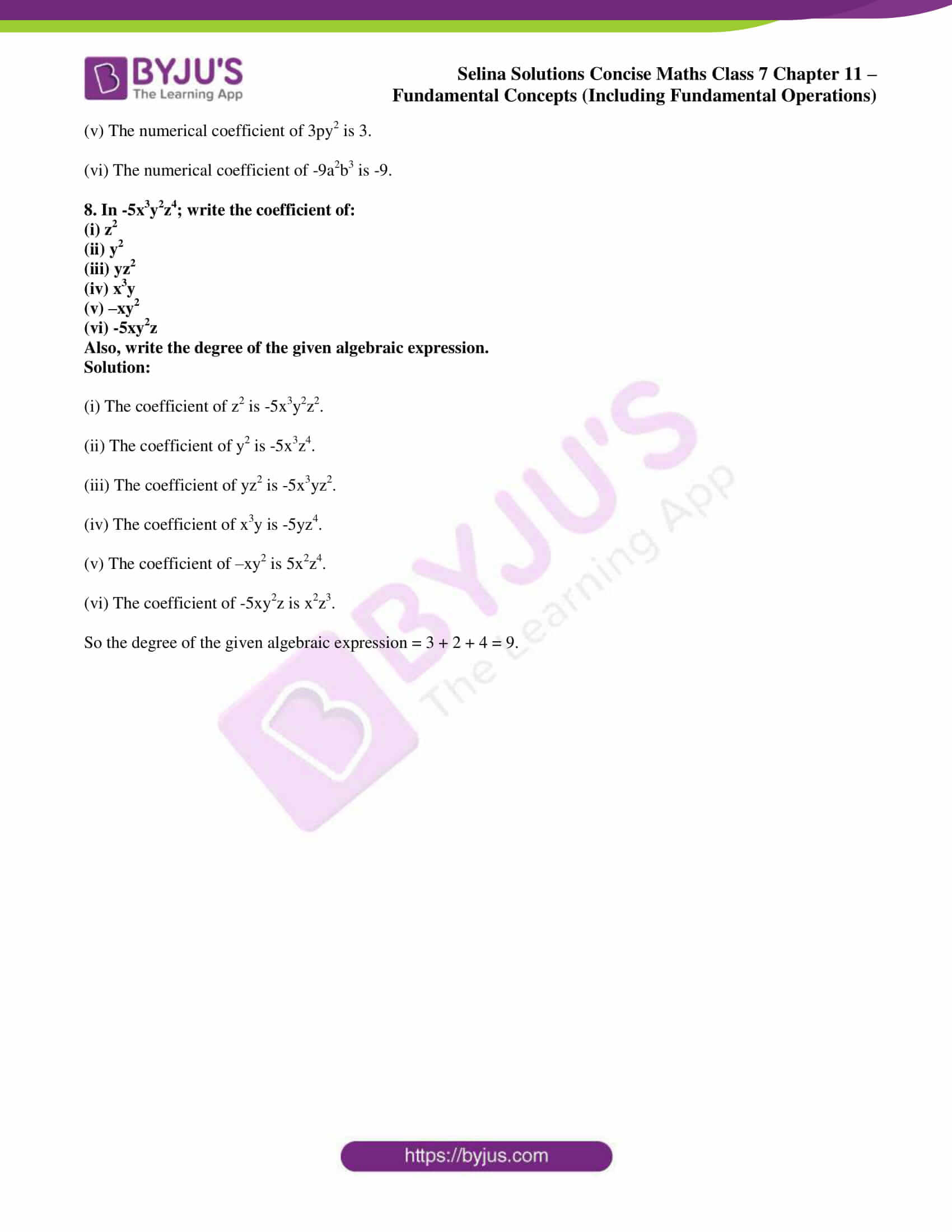# Selina Solutions Concise Maths Class 7 Chapter 11: Fundamental Concepts (Including Fundamental Operations) Exercise 11A

Selina Solutions Concise Maths Class 7 Chapter 11 Fundamental Concepts (Including Fundamental Operations) Exercise 11A provides students with a clear picture of the basic terms, which are important from the exam perspective. The solutions contain concepts explained in a simple language to help students perform well in the annual exam. The expert faculty at BYJU’S design the solutions after conducting vast research on each concept. Students can refer to Selina Solutions Concise Maths Class 7 Chapter 11 Fundamental Concepts (Including Fundamental Operations) Exercise 11A PDF, from the links provided here.

## Selina Solutions Concise Maths Class 7 Chapter 11: Fundamental Concepts (Including Fundamental Operations) Exercise 11A Download PDF### Access other exercises of Selina Solutions Concise Maths Class 7 Chapter 11: Fundamental Concepts (Including Fundamental Operations)

Exercise 11B Solutions

Exercise 11C Solutions

Exercise 11D Solutions

Exercise 11E Solutions

Exercise 11F Solutions

### Access Selina Solutions Concise Maths Class 7 Chapter 11: Fundamental Concepts (Including Fundamental Operations) Exercise 11A

#### Exercise 11A page: 121

1. Separate constant terms and variable terms from the following:

8, x, 6xy, 6 + x, – 5xy2, 15az2, 32z/ xy, y2/ 3x

Solution:

The constant term is 8.

The variable terms are x, 6xy, 6 + x, – 5xy2, 15az2, 32z/ xy, y2/ 3x.

2. For each expression, given below, state whether it is a monomial, binomial or trinomial:

(i) 2x ÷ 15

(ii) ax + 9

(iii) 3x2 × 5x

(iv) 5 + 2x – 3b

(v) 2y – 7z/3 ÷ x

(vi) 3p × q ÷ z

(vii) 12z ÷ 5x + 4

(viii) 12 – 5z – 4

(ix) a3 -3ab2 × c

Solution:

(i) 2x ÷ 15 = 2x/15

It has one term and hence it is a monomial.

(ii) ax + 9

It has two terms and hence it is a binomial.

(iii) 3x2 × 5x = 15x3

It has one term and hence it is a monomial.

(iv) 5 + 2x – 3b

It has three terms and hence it is a trinomial.

(v) 2y – 7z/3 ÷ x = 2y – 7z/3x

It has two terms and hence it is a binomial.

(vi) 3p × q ÷ z = 3pq/z

It has one term and hence it is a monomial.

(vii) 12z ÷ 5x + 4 = 12z/5x + 4

It has two terms and hence it is a binomial.

(viii) 12 – 5z – 4 = 8 – 5z

It has two terms and hence it is a binomial.

(ix) a3 -3ab2 × c = a3 – 3ab2c

It has two terms and hence it is a binomial.

3. Write the coefficient of:

(i) xy in -3axy

(ii) z2 in p2yz2

(iii) mn in – mn

(iv) 15 in -15p2

Solution:

(i) xy in -3axy

The coefficient of xy in -3axy = -3a

(ii) z2 in p2yz2

The coefficient of z2 in p2yz2 = p2y

(iii) mn in – mn

The coefficient of mn in – mn = -1

(iv) 15 in -15p2

The coefficient of 15 in -15p2 = -p2

4. For each of the following monomials, write its degree:

(i) 7y

(ii) –x2y

(iii) xy2z

(iv) -9y2z3

(v) 3m3n4

(vi) -2p2q3r4

Solution:

(i) The degree of 7y is 1.

(ii) The degree of –x2y = 2 + 1 = 3

(iii) The degree of xy2z = 1 + 2 + 1 = 4

(iv) The degree of -9y2z3 = 2 + 3 = 5

(v) The degree of 3m3n4 = 3 + 4 = 7

(vi) The degree of -2p2q3r4 = 2 + 3 + 4 = 9

5. Write the degree of each of the following polynomials:

(i) 3y3 – x2y2 + 4x

(ii) p3q2 – 6p2q5 + p4q4

(iii) -8mn6 + 5m3n

(iv) 7 – 3x2y + y2

(v) 3x – 15

(vi) 2y2z + 9yz3

Solution:

(i) The degree of 3y3 – x2y2 + 4x is 4

x2y2 is the term which has the highest degree.

(ii) The degree of p3q2 – 6p2q5 + p4q4 is 8

p4q4 is the term which has the highest degree.

(iii) The degree of -8mn6 + 5m3n is 7

-8mn6 is the term which has the highest degree.

(iv) The degree of 7 – 3x2y + y2 is 3

– 3x2y is the term which has the highest degree.

(v) The degree of 3x – 15 is 1

3x is the term which has the highest degree.

(vi) The degree of 2y2z + 9yz3 is 4

9yz3 is the term which has the highest degree.

6. Group the like terms together:

(i) 9x2, xy, -3x2, x2 and -2xy

(ii) ab, -a2b, -3ab, 5a2b and -8a2b.

(iii) 7p, 8pq, -5pq, -2p and 3p

Solution:

(i) 9x2, xy, -3x2, x2 and -2xy

9x2, -3x2 and x2 are like terms

xy and -2xy are like terms.

(ii) ab, -a2b, -3ab, 5a2b and -8a2b

-a2b, 5a2b and -8a2b are like terms

ab and – 3ab are like terms.

(iii) 7p, 8pq, -5pq, -2p and 3p

7p, -2p and 3p are like terms

8pq and -5pq are like terms.

7. Write the numerical coefficient of each of the following:

(i) y

(ii) – y

(iii) 2x2y

(iv) -8xy3

(v) 3py2

(vi) -9a2b3

Solution:

(i) The numerical coefficient of y is 1.

(ii) The numerical coefficient of – y is – 1.

(iii) The numerical coefficient of 2x2y is 2.

(iv) The numerical coefficient of -8xy3 is -8.

(v) The numerical coefficient of 3py2 is 3.

(vi) The numerical coefficient of -9a2b3 is -9.

8. In -5x3y2z4; write the coefficient of:

(i) z2

(ii) y2

(iii) yz2

(iv) x3y

(v) –xy2

(vi) -5xy2z

Also, write the degree of the given algebraic expression.

Solution:

(i) The coefficient of z2 is -5x3y2z2.

(ii) The coefficient of y2 is -5x3z4.

(iii) The coefficient of yz2 is -5x3yz2.

(iv) The coefficient of x3y is -5yz4.

(v) The coefficient of –xy2 is 5x2z4.

(vi) The coefficient of -5xy2z is x2z3.

So the degree of the given algebraic expression = 3 + 2 + 4 = 9.